# R语言广义相加模型 (GAMs)分析预测CO2时间序列数据

## 环境科学中的许多数据不适合简单的线性模型，最好用广义相加模型（GAM）来描述。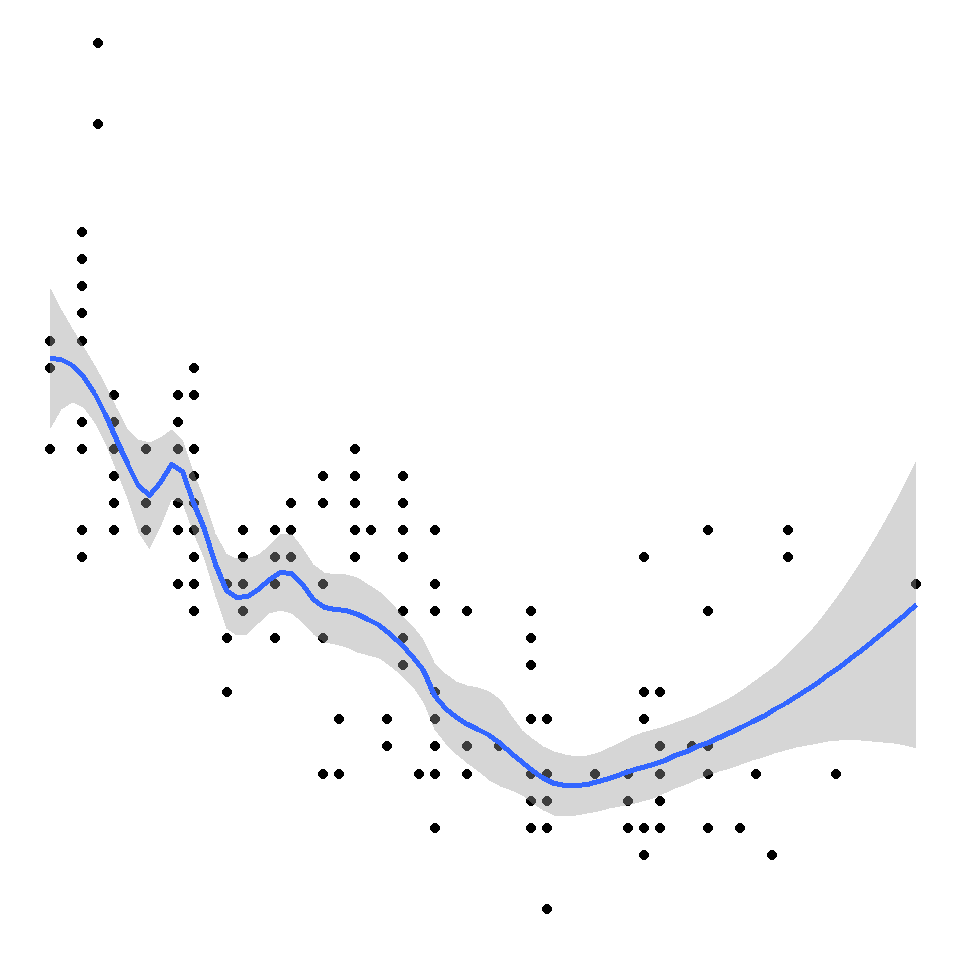# 理论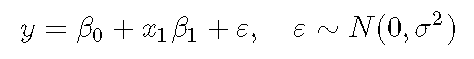GAM中发生的变化是存在光滑项：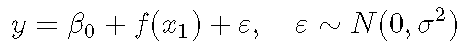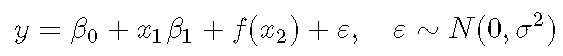R语言广义相加模型（GAM）在电力负荷预测中的应用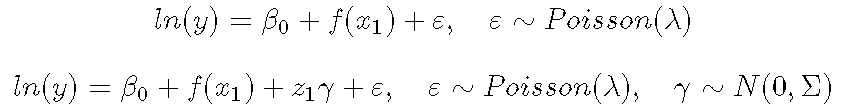# 一个简单的例子

``````x <- seq(0, pi * 2, 0.1)
sin_x <- sin(x)
y <- sin_x + rnorm(n = length(x), mean = 0, sd = sd(sin_x / 2))
Sample <- data.frame(y,x)``````
``````library(ggplot2)
ggplot(Sample, aes(x, y)) + geom_point()``````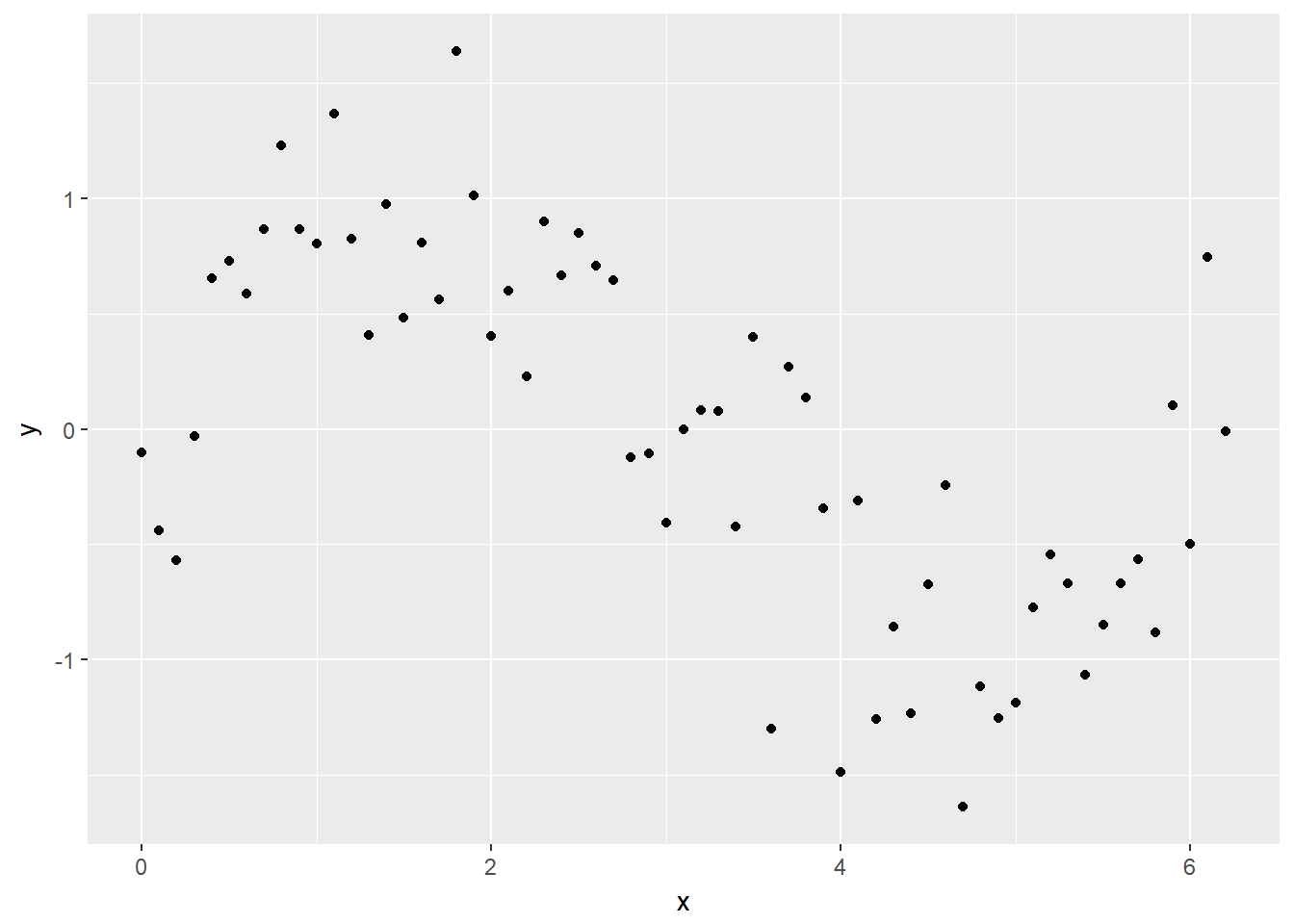# 在r语言中使用GAM（广义相加模型）进行电力负荷时间序列分析

``lm_y <- lm(y ~ x, data = Sample)``

``ggplot(Sample, aes(x, y)) + geom_point() + geom_smooth(method = lm)``

### 随时关注您喜欢的主题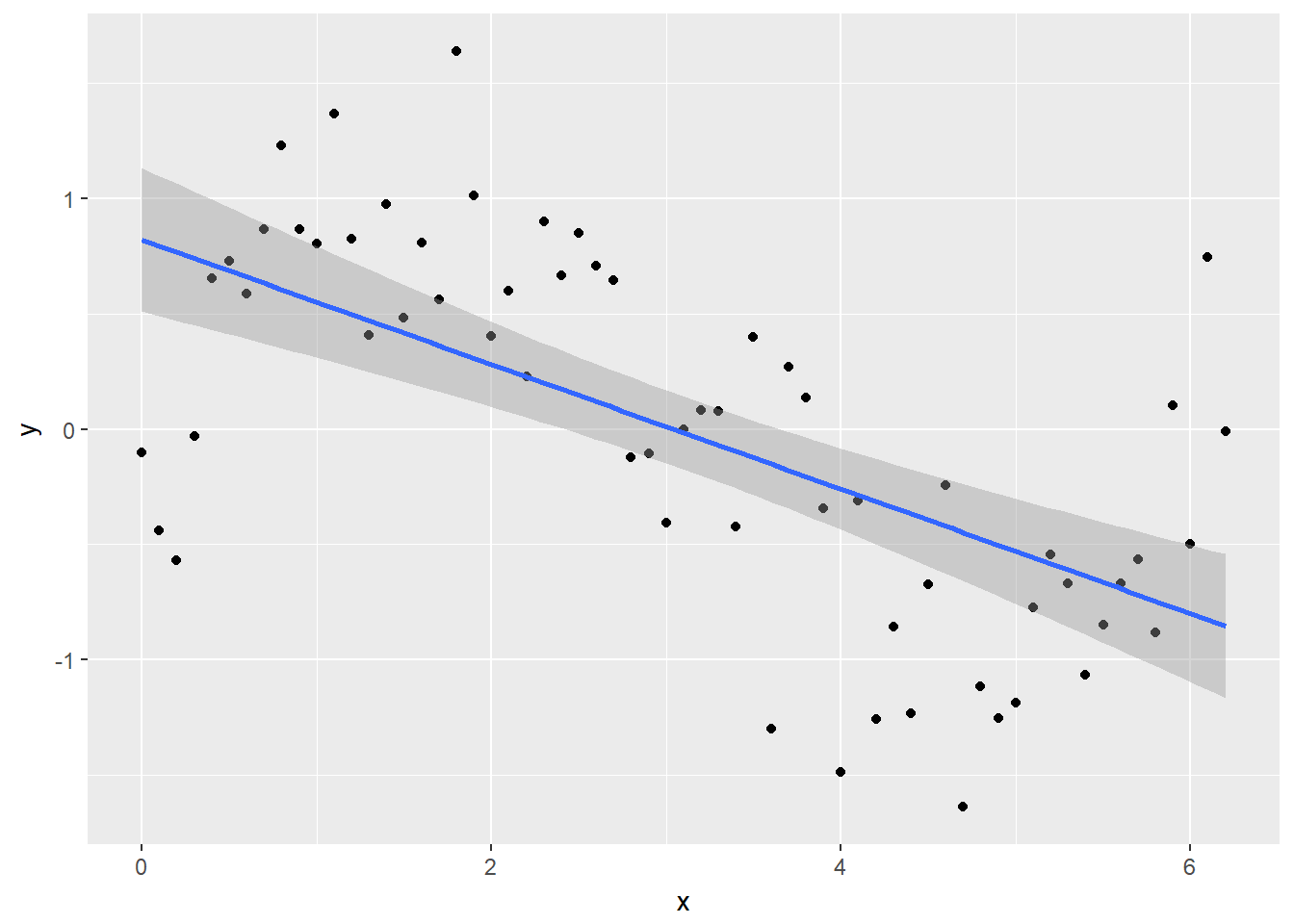``plot(lm_y, which = 1)``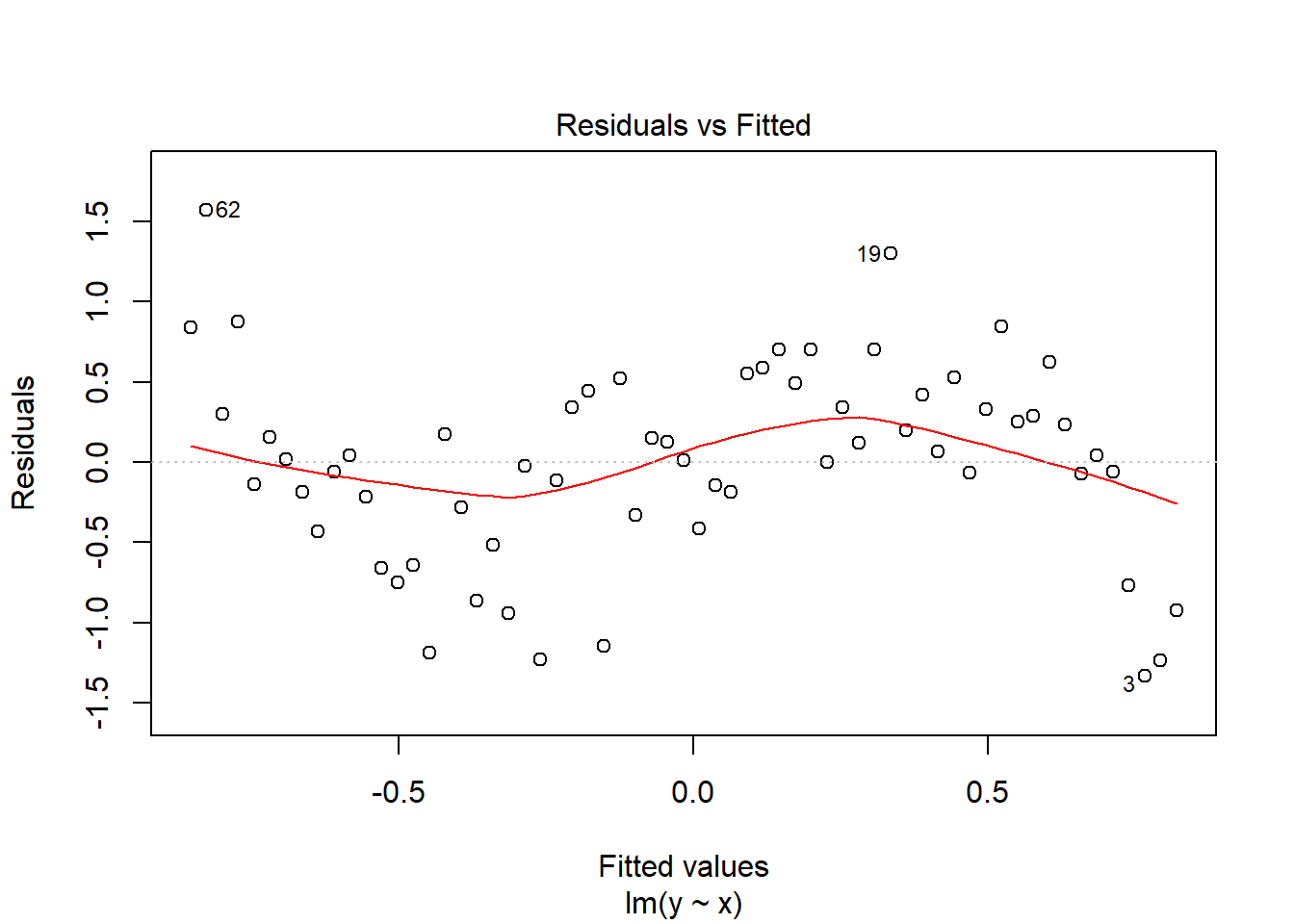# 运行分析

``gam_y <- gam(y ~ s(x), method = "REML")``

``predict(gam_y, data.frame(x = x_new))``

# 但是对于简单的模型，我们还可以利用中的 `method =` 参数来 `geom_smooth`指定模型公式。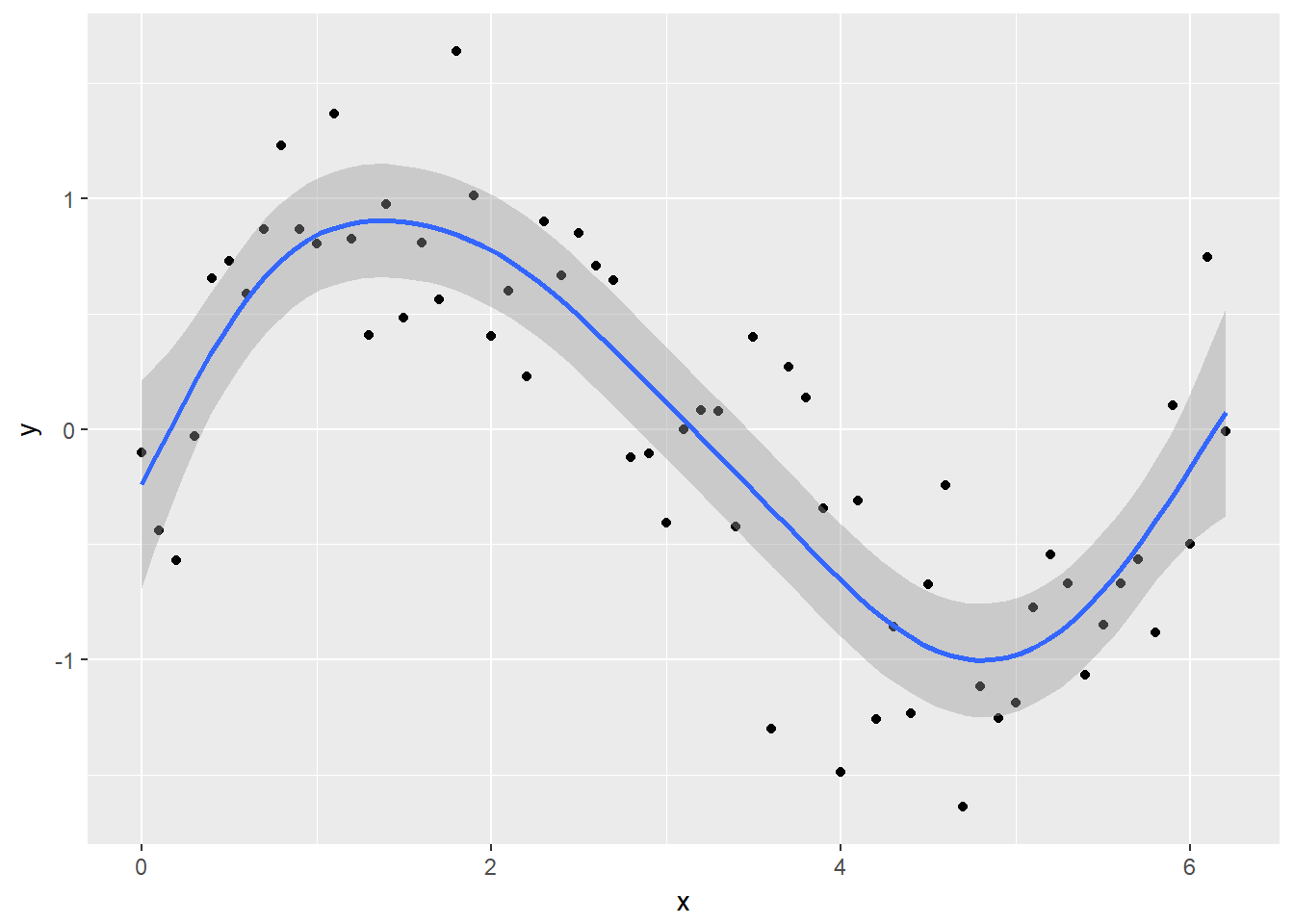`check.gam` 快速简便地查看残差图。

``gam.check(gam_y)``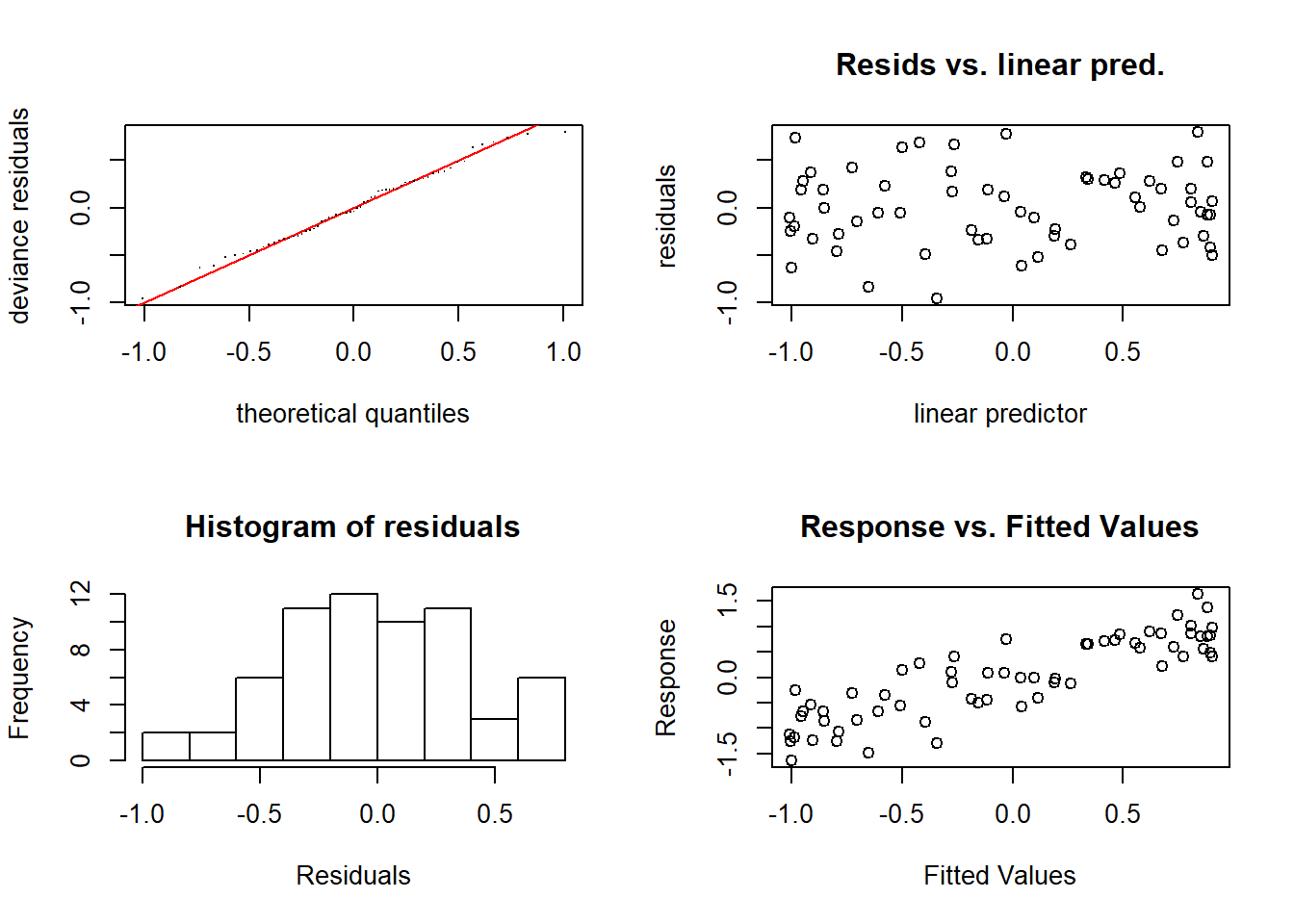# 对模型对象使用summary将为您提供光滑项（以及任何参数项）的意义，以及解释的方差。

``````##
## Method: REML   Optimizer: outer newton
## full convergence after 6 iterations.
## (score 44.14634 & scale 0.174973).
## Hessian positive definite, eigenvalue range [1.75327,30.69703].
## Model rank =  10 / 10
##
## Basis dimension (k) checking results. Low p-value (k-index<1) may
## indicate that k is too low, especially if edf is close to k'.
##
##        k'  edf k-index p-value
## s(x) 9.00 5.76    1.19     0.9``````

``````##
## Family: gaussian
##
## Formula:
## y ~ s(x)
##
## Parametric coefficients:
##             Estimate Std. Error t value Pr(>|t|)
## (Intercept) -0.01608    0.05270  -0.305    0.761
##
## Approximate significance of smooth terms:
##       edf Ref.df     F p-value
## s(x) 5.76  6.915 23.38  <2e-16 ***
## ---
## Signif. codes:  0 '***' 0.001 '**' 0.01 '*' 0.05 '.' 0.1 ' ' 1
##
## R-sq.(adj) =  0.722   Deviance explained = 74.8%
## -REML = 44.146  Scale est. = 0.17497   n = 63``````

# 基函数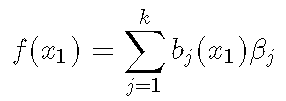``````model_matrix <- predict(gam_y, type = "lpmatrix")
plot(y ~ x) ``````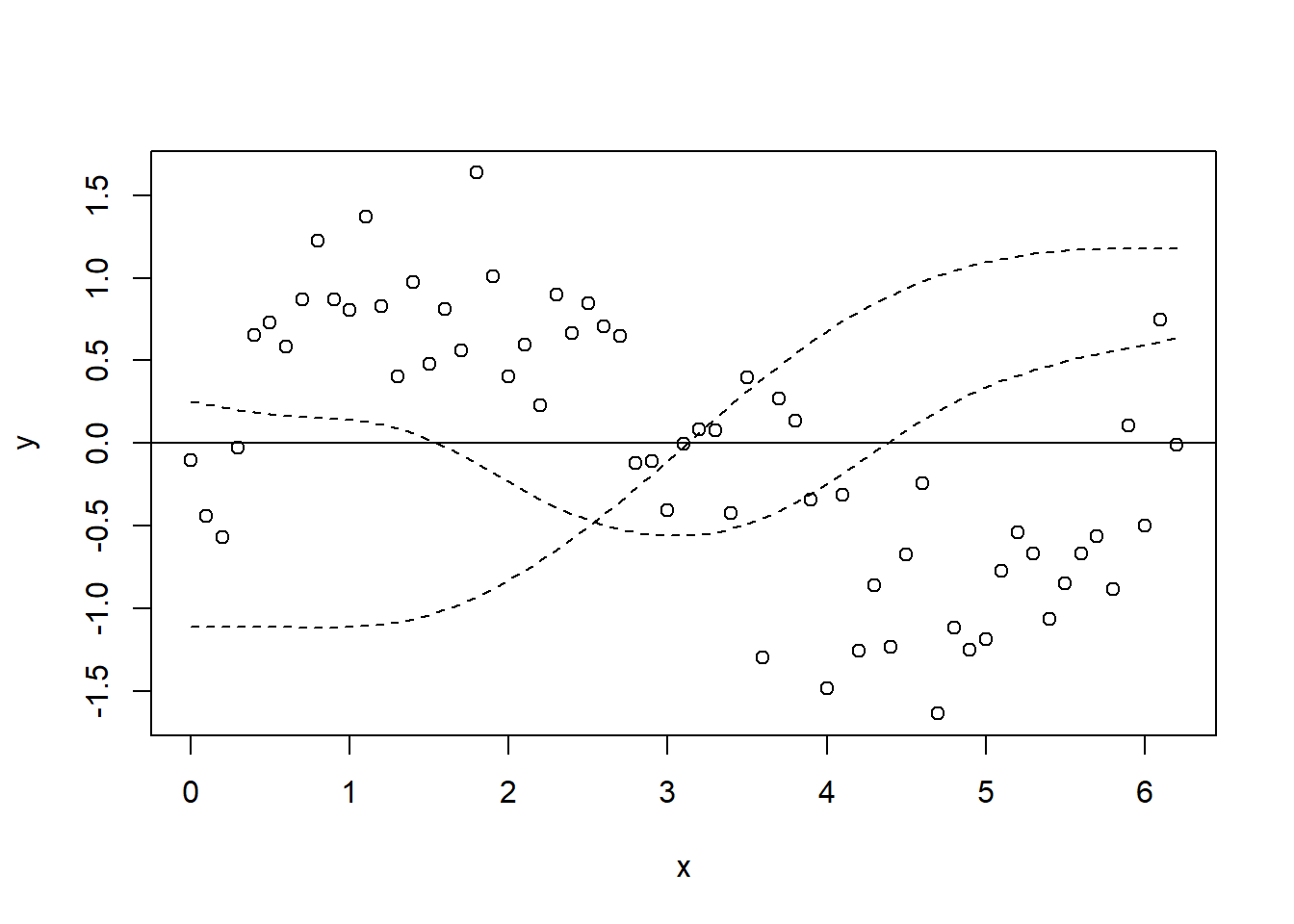`````` matplot(x, model_matrix[,-1], type = "l", lty = 2, add = T)
lines(y_pred ~ x_new, col = "red", lwd = 2)``````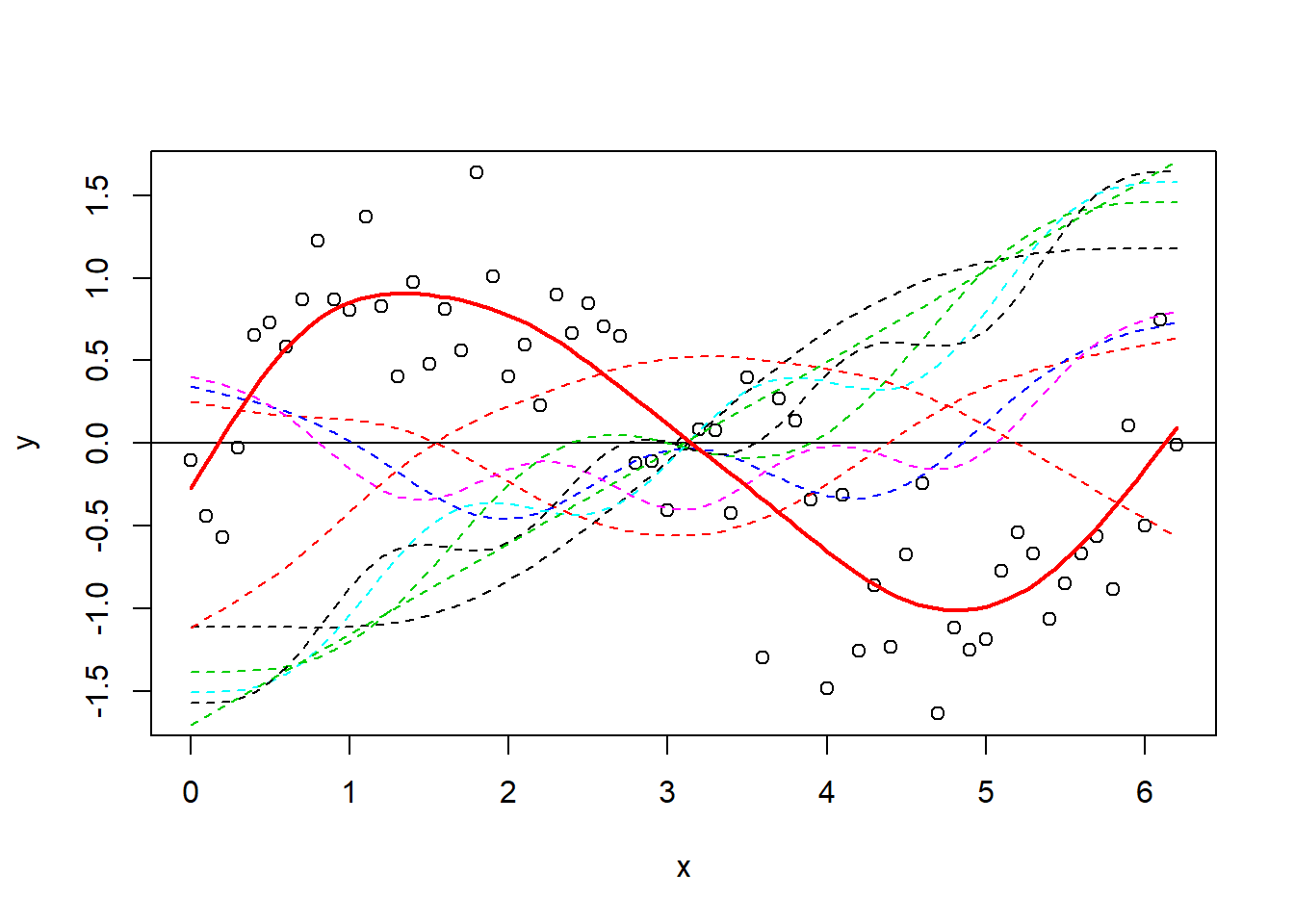# 现在，让我们绘制所有基函数的图，然后再将其添加到GAM（`y_pred`）的预测中。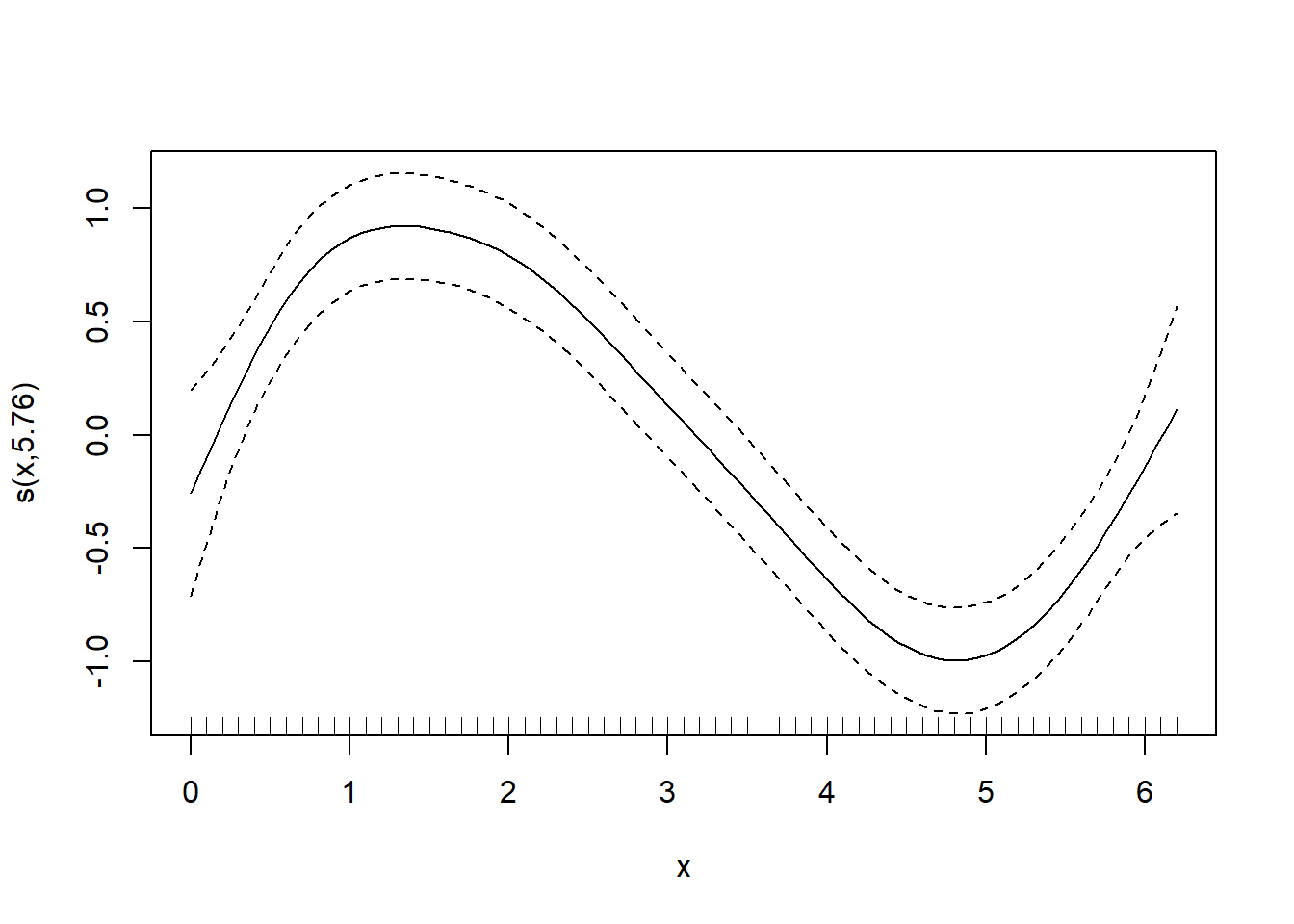``x_sin_smooth <- smoothCon(s(x), data = data.frame(x), absorb.cons = TRUE) ``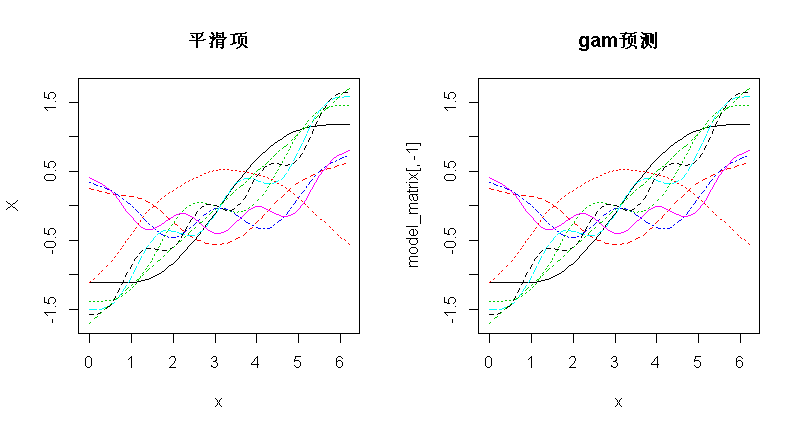``````betas <- gam_y\$coefficients
linear_pred <- model_matrix %*% betas ``````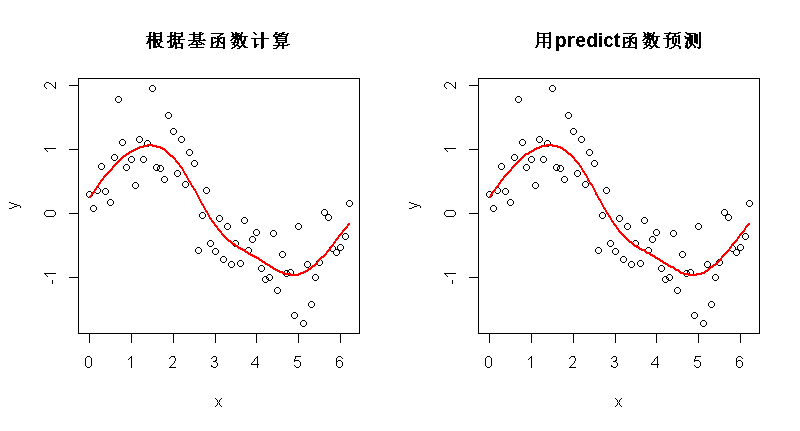# 真实例子``CO2 <- read.csv("co2.csv")``

``CO2\$time <- as.integer(as.Date(CO2\$Date, format = "%d/%m/%Y")) ``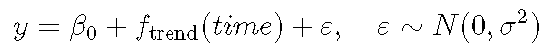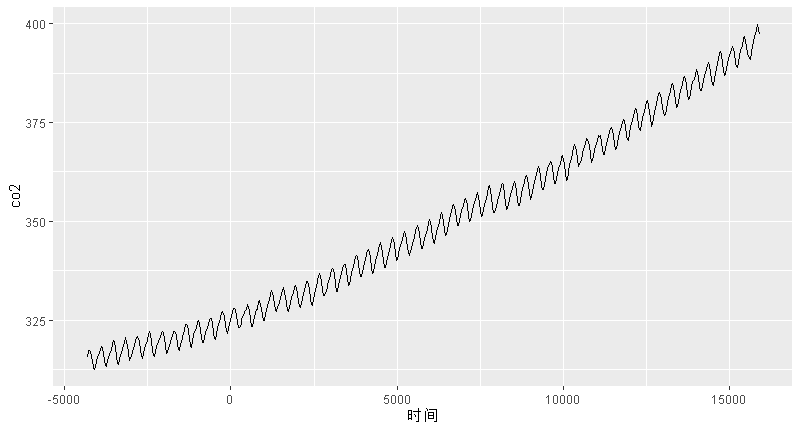``plot(CO2_time)``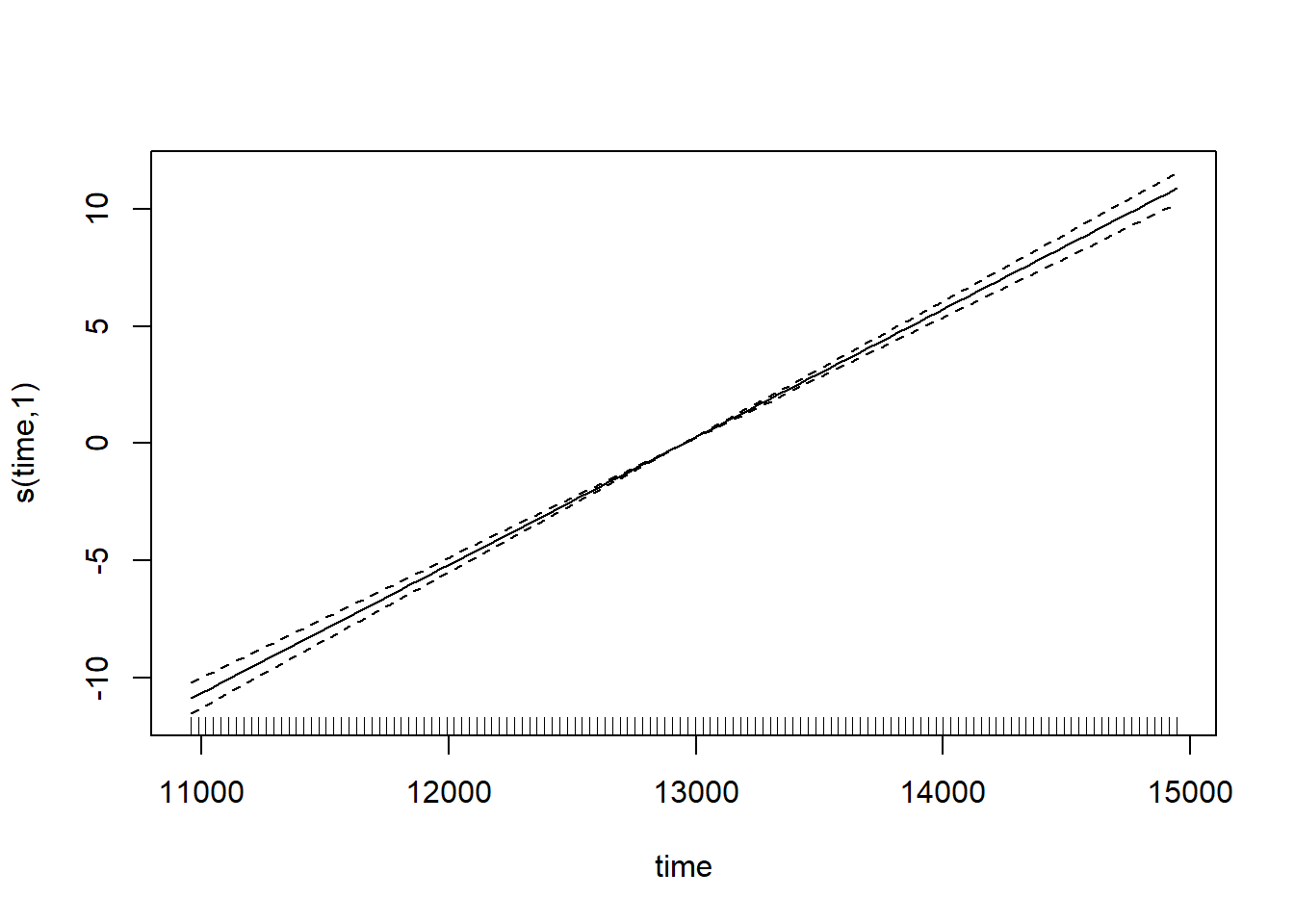``````par(mfrow = c(2,2))
gam.check(CO2_time)``````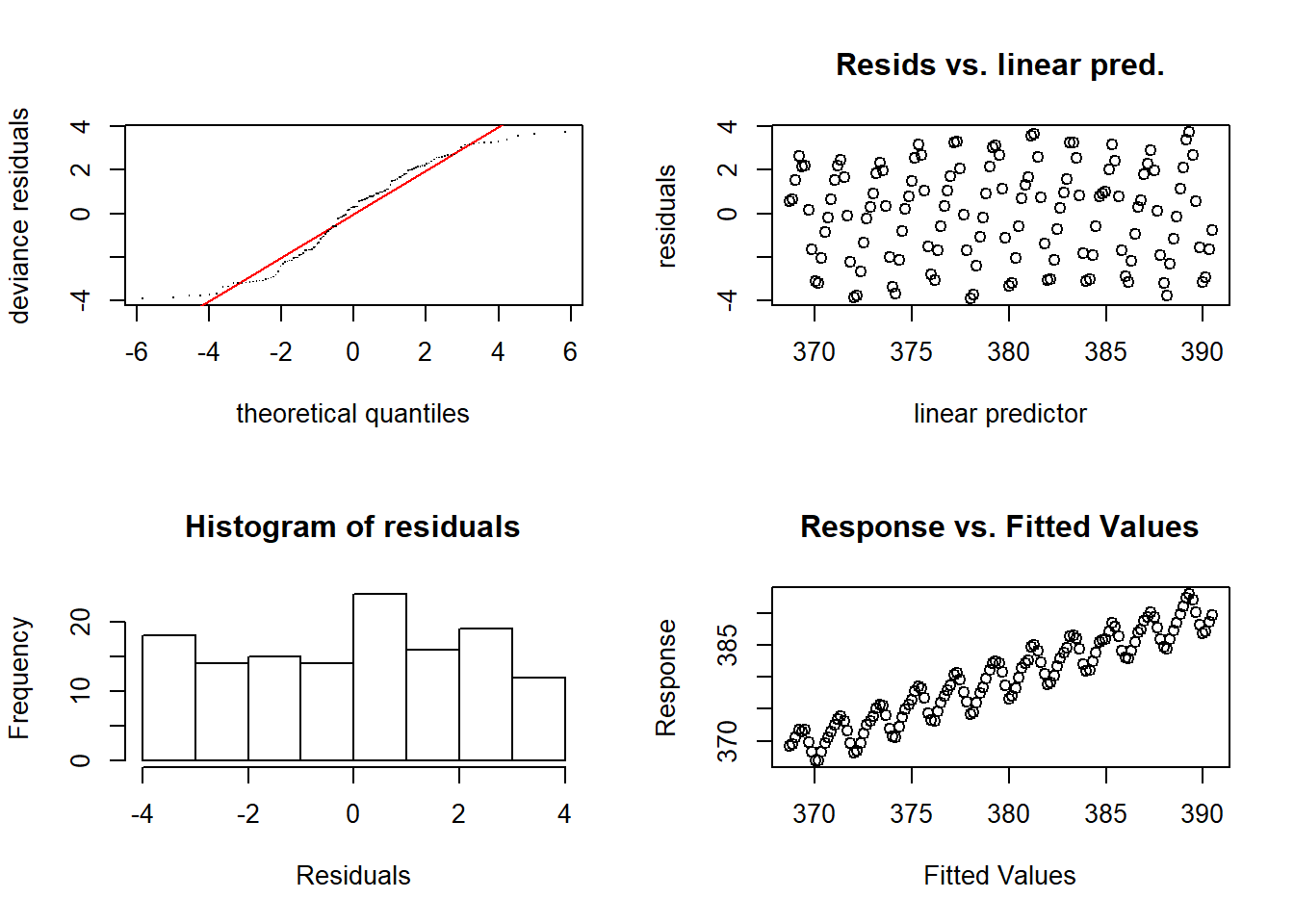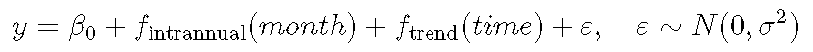``s(month, bs = 'cc', k = 12) + s(time)``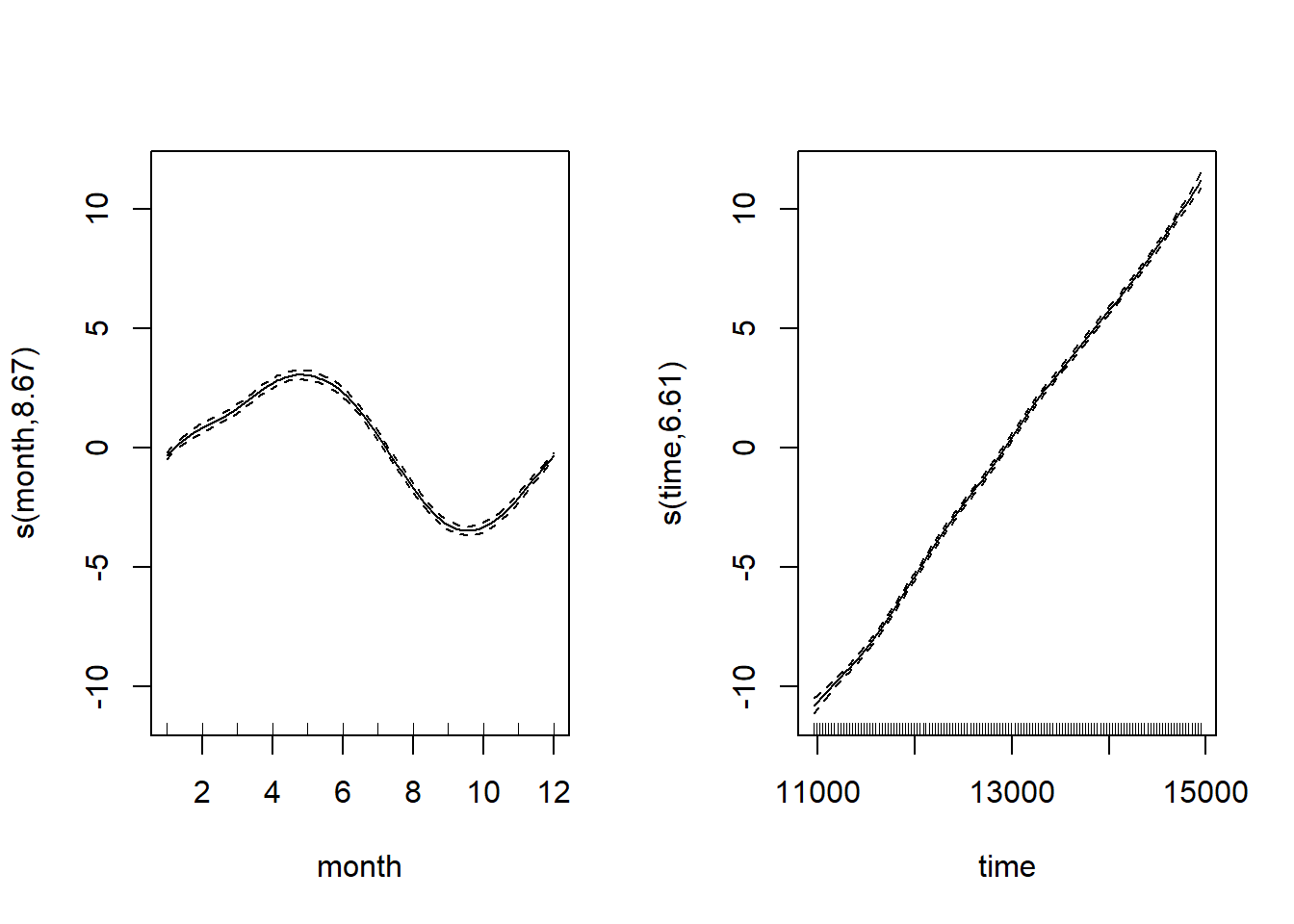``````par(mfrow = c(2,2))
gam.check(CO2_season_time)``````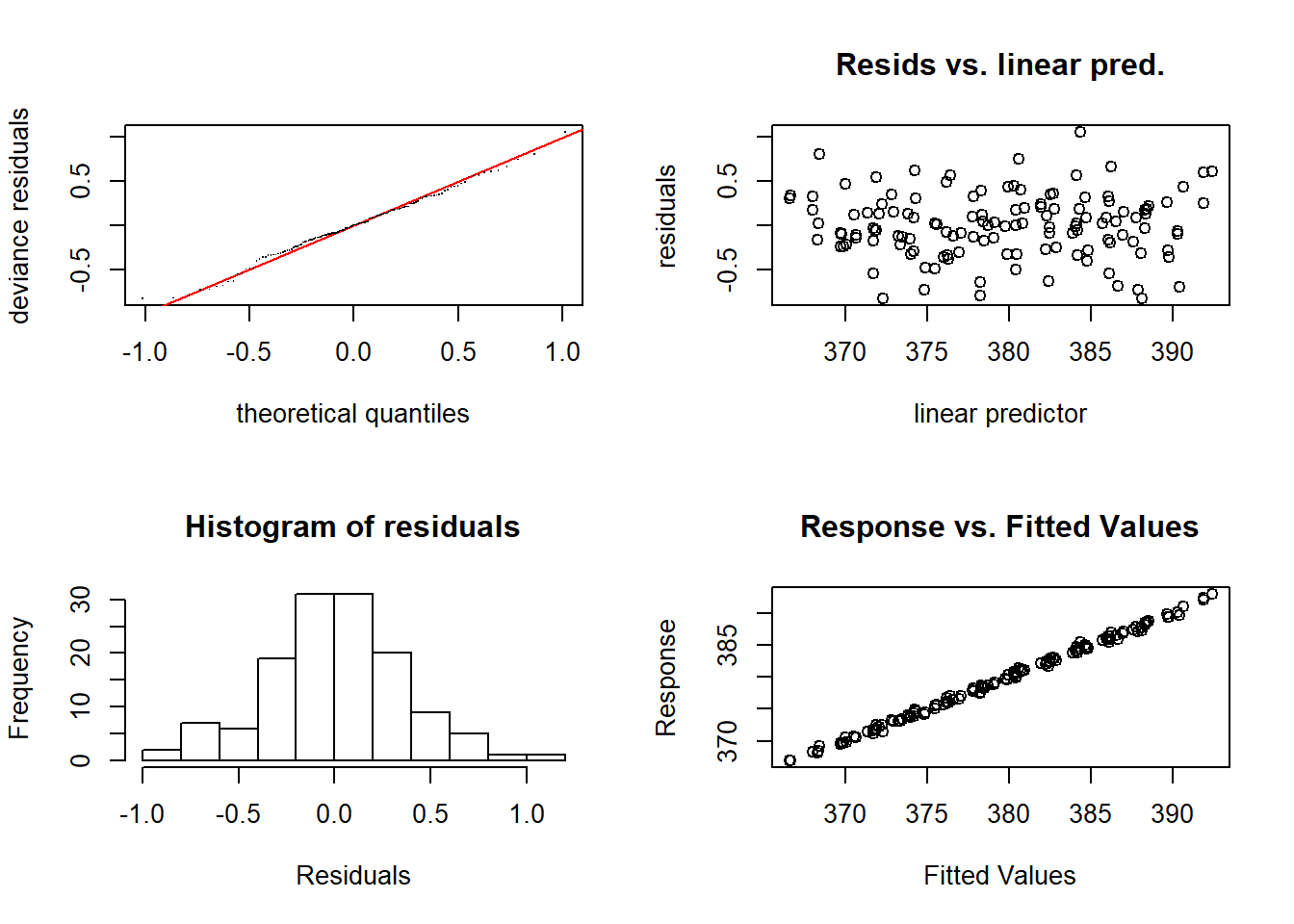`` plot(CO2_season_time)``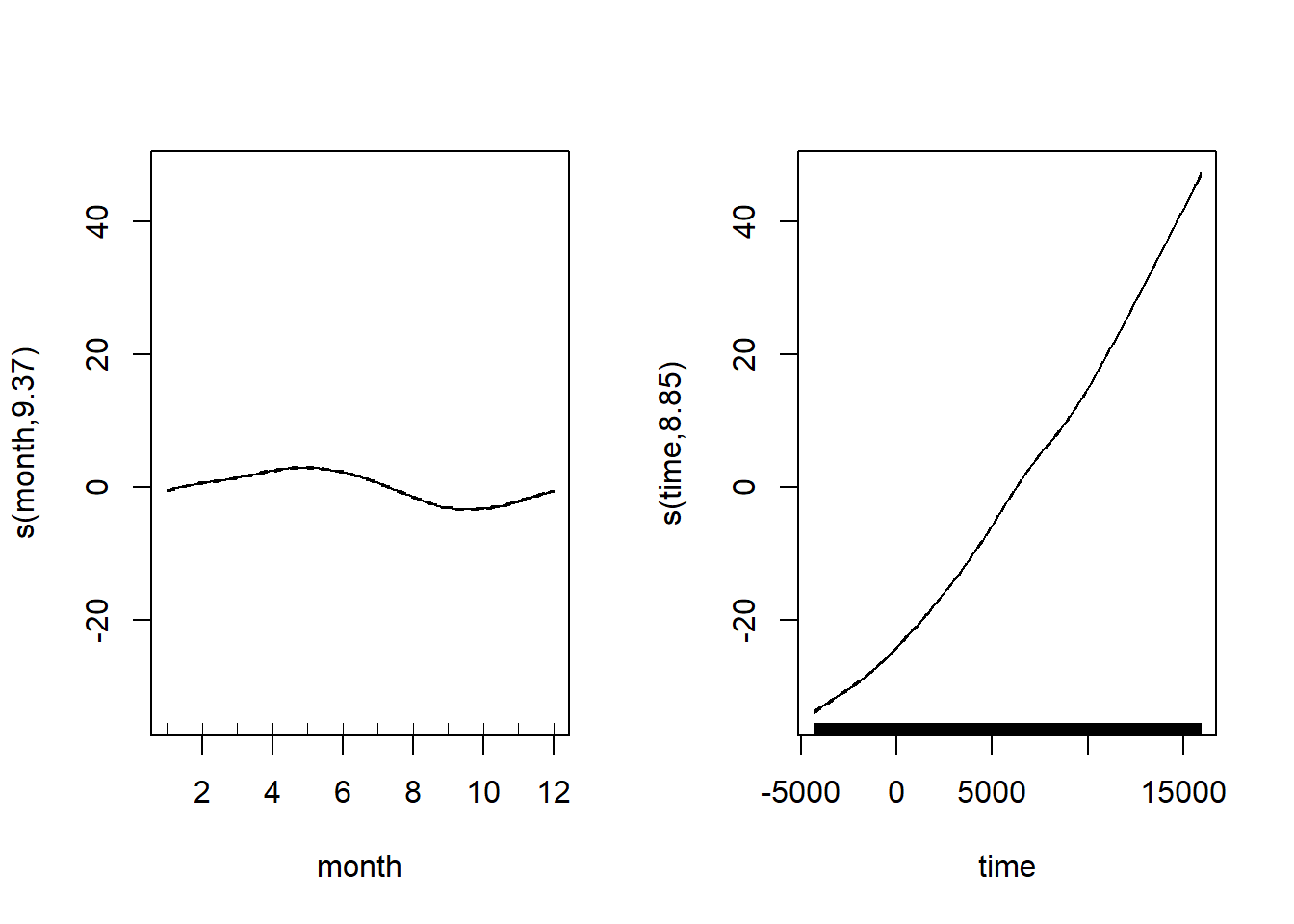# 结果

``geom_line(aes(y = predicted_values)``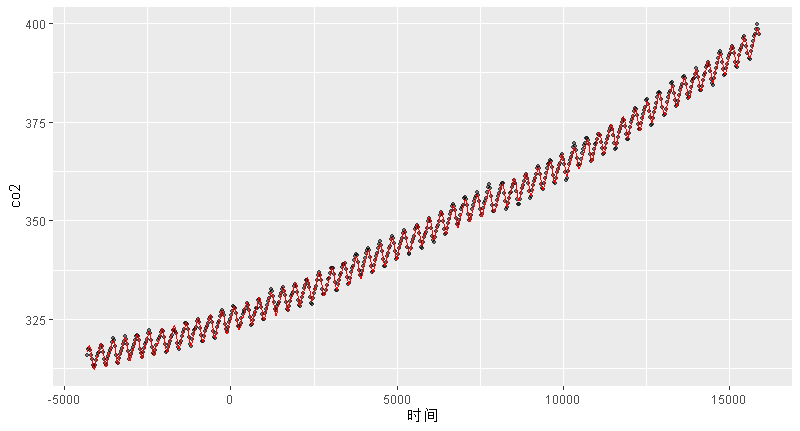Kaizong Ye拓端研究室（TRL）的研究员。

​非常感谢您阅读本文，如需帮助请联系我们！QQ在线咨询

15121130882

0571-63341498

## 关注有关新文章的微信公众号

This will close in 0 seconds